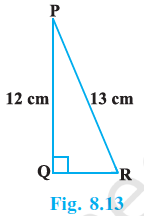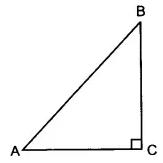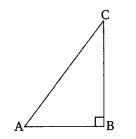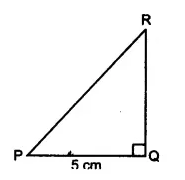# NCERT Solutions for Class 10 Maths Chapter 8 Introduction to Trigonometry

NCERT solutions for class 10 maths chapter 8 Introduction to Trigonometry - The chapter trigonometry has been generated from the right angle triangle. The relation between the angles and sides of a right angle triangle is called trigonometry. In solutions of NCERT class 10 maths chapter 8 Introduction to Trigonometry, there is a detailed explanation for each question of practice exercise. In this particular chapter, you will study some ratios of a right-angled triangle with respect to its acute angles, and this ratio is called a trigonometric ratio of the angles. CBSE NCERT solutions for class 10 maths chapter 8 Introduction to Trigonometry is also covering the questions based on trigonometric ratios. Unit 5 "Trigonometry" holds 12 marks out of 80 marks in the maths paper of CBSE board examination and we can expect 2-3 questions from this chapter of total around 8 marks. There is a total of 4 exercises with 27 questions in the entire chapter. NCERT solutions for class 10 maths chapter 8 Introduction to Trigonometry are designed to provide assistance for homework and for preparing the board examinations. If you want to download the NCERT solutions for free then you can have it by clicking on the link.

The trigonometric ratios of the angle A in right triangle ABC are defined as follows-The values of all the trigonometric ratios of 0°, 30°, 45°, 60°, and 90° are-

 Sin A 0 1 Cos A 1 0 Tan A 0 1 Not defined Cosec A Not defined 2 1 Sec A 1 2 Not defined Cot A Not defined 1 0

## NCERT solutions for class 10 maths chapter 8 Introduction to Trigonometry Excercise: 8.1

### Q1 In , right-angled at , . Determine :We have,
In B = 90, and the length of the base (AB) = 24 cm and length of perpendicular (BC) = 7 cm
So, by using Pythagoras theorem,

Therefore,

AC = 25 cm

Now,
(i)

(ii)  For angle C, AB is perpendicular to the base (BC).  Here B  indicates to Base and P means perpendicular wrt angle C
So,
andWe have, PQR is a right-angled triangle, length of PQ and PR are 12 cm and 13 cm respectively.
So, by using Pythagoras theorem,

Now, According to question,
=
= 5/12 - 5/12  = 0

Suppose ABC is a right-angled triangle in which  and we have
So,Let the length of AB be 4 unit and the length of BC = 3 unit
So, by using Pythagoras theorem,
units
Therefore,
and

We have,

It implies that In the triangle ABC in which . The length of AB be 8 units and the length of BC  = 15 units

Now, by using Pythagoras theorem,

units

So,
and

We have,

It means the Hypotenuse of the triangle is 13 units and the base is 12 units.
Let ABC is a right-angled triangle in which B is 90 and AB is the base, BC is perpendicular height and AC is the hypotenuse.By using Pythagoras theorem,

BC = 5 unit

Therefore,

We have, A and B are two acute angles of triangle ABC andAccording to question,
In triangle ABC,

Therefore, A =  B   [angle opposite to equal sides are equal]

Given that,

perpendicular  (AB) = 8 units and Base (AB) = 7 units
Draw a right-angled triangle ABC in which
Now, By using Pythagoras theorem,

So,
and

Given that,

ABC is a right-angled triangle in which  and the length of the base AB is 4 units and length of perpendicular is 3 unitsBy using Pythagoras theorem,
In triangle ABC,

AC = 5 units

So,

Put the values of above trigonometric ratios, we get;

LHS  RHS

Given a triangle ABC, right-angled at  B andAccording to question,

By using Pythagoras theorem,

AC = 2
Now,

Therefore,We have,
PR + QR = 25 cm.............(i)
PQ = 5 cm
and
According to question,
In triangle PQR,
By using Pythagoras theorem,

PR - QR = 1........(ii)

From equation(i) and equation(ii), we get;
PR = 13 cm  and QR = 12 cm.

therefore,

(i) The value of is always less than 1.
(ii)  for some value of angle A.
(iii) is the abbreviation used for the cosecant of angle A.
(iv) is the product of cot and A.
(v)   for some angle

(i) False,
because , which is greater than 1

(ii) TRue,
because

(iii) False,
Because  abbreviation is used for cosine A.

(iv) False,
because the term  is a single term, not a product.

(v) False,
because  lies between (-1 to +1) []

## NCERT solutions for class 10 maths chapter 8 Introduction to Trigonometry Excercise: 8.2

As we know,
the value of  ,

We know the value of

and

According to question,

## Q1 Evaluate the following :

we know the value of

,  and ,

After putting these values

..................(i)
It is known that the values of the given trigonometric functions,

Put all these values in equation (i), we get;

.....................(i)
We know the values of-

By substituting all these values in equation(i), we get;

Put the value of tan 30 in the given question-

The correct option is (A)

The correct option is (D)

We know that
So,

is true when =

The correct option is (A)

We know that
So,

Put the value of

The correct option is (C)

### Q3 If  and   find

Given that,

So, ..........(i)

therefore, .......(ii)
By solving the equation (i) and (ii) we get;

and

The value of   increases as  increases.
The value of  increases as  increases.
for all values of  .
is not defined for

(i) False,
Let A = B =
Then,

(ii) True,
Take
whent
= 0 then zero(0),
= 30 then value of  is 1/2 = 0.5
= 45 then value of  is 0.707

(iii) False,

(iv) False,
Let  = 0

(v) True,
(not defined)

## NCERT solutions for class 10 maths chapter 8 Introduction to Trigonometry Excercise: 8.3

We can write the above equation as;

By using the identity of
Therefore,

The above equation can be written as ;

.........(i)
It is known that,
Therefore, equation (i) becomes,

The above equation can be written as ;
....................(i)
It is known that
Therefore, equation (i) becomes,

This equation can be written as;
.................(i)
We know that

Therefore, equation (i) becomes;
= 0

Taking Left Hand Side (LHS)

[it is known that  and

Hence proved.

Taking Left Hand Side (LHS)
=
=
= [it is known that  and  ]
= 0

We have,
2A  =  (A -
we know that,

We have,

and we know that
therefore,

A = 90 - B
A + B = 90
Hence proved.

We have,
, Here 4A is an acute angle
According to question,
We know that

Given that,
A, B and C are interior angles of
To prove -

Now,
In triangle
A + B + C  =

Hence proved.

By using the identity of  and

We know that,
and
the above equation can be written as;

## NCERT Solutions for Class 10 Maths Chapter 8 Introduction to Trigonometry Excercise: 8.4

We know that
(i)

(ii) We know the identity of

(iii)

We know that the identity

....................(i)

The above equation can be written as;

(Since )

We know that

Therefore,

(A) 1    (B) 9    (C) 8    (D) 0

The correct option is (B) = 9

.............(i)

and it is known that
Therefore, equation (i) becomes,

(A) 0    (B) 1    (C) 2    (D) –1

The correct option is (C)

.......................(i)

we can write his above equation as;

= 2

The correct option is (D)

The correct option is (D)

..........................eq (i)

The above equation can be written as;

We know that

therefore,

We need to prove-

Now, taking LHS,

LHS = RHS

Hence proved.

We need to prove-

taking LHS;

= RHS

Hence proved.

[Hint : Write the expression in terms of and ]

We need to prove-

Taking LHS;

By using the identity a3 - b3 =(a - b) (a2 + b2+ab)

Hence proved.

[Hint : Simplify LHS and RHS separately]

We need to prove-

taking LHS;

Taking RHS;
We know that identity

LHS = RHS

Hence proved.

## Q5 Prove the following identities, where the angles involved are acute angles for which the expressions are defined.  , using the identity

We need to prove -

Dividing the numerator and denominator by , we get;

Hence Proved.

We need to prove -

Taking LHS;
By rationalising the denominator, we get;

Hence proved.

We need to prove -

Taking LHS;
[we know the identity ]

Hence proved.

Given equation,
..................(i)

Taking LHS;

[since ]

Hence proved

[Hint : Simplify LHS and RHS separately]

We need to prove-

Taking LHS;

Taking RHS;

LHS = RHS

Hence proved.

We need to prove,

Taking LHS;

Taking RHS;

LHS = RHS

Hence proved.

## NCERT solutions for class 10 maths chapter wise

 Chapter No. Chapter Name Chapter 1 CBSE NCERT solutions for class 10 maths chapter 1 Real Numbers Chapter 2 NCERT solutions for class 10 maths chapter 2 Polynomials Chapter 3 Solutions of NCERT class 10 maths chapter 3 Pair of Linear Equations in Two Variables Chapter 4 CBSE NCERT solutions for class 10 maths chapter 4 Quadratic Equations Chapter 5 NCERT solutions for class 10 chapter 5 Arithmetic Progressions Chapter 6 Solutions of NCERT class 10 maths chapter 6 Triangles Chapter 7 CBSE NCERT solutions for class 10 maths chapter 7 Coordinate Geometry Chapter 8 NCERT solutions for class 10 maths chapter 8 Introduction to Trigonometry Chapter 9 Solutions of NCERT class 10 maths chapter 9 Some Applications of Trigonometry Chapter 10 CBSE NCERT solutions class 10 maths chapter 10 Circles Chapter 11 NCERT solutions  for class 10 maths chapter 11 Constructions Chapter 12 Solutions of NCERT class 10 chapter maths chapter 12 Areas Related to Circles Chapter 13 CBSE NCERT solutions class 10 maths chapter 13 Surface Areas and Volumes Chapter 14 NCERT solutions for class 10 maths chapter 14 Statistics Chapter 15 Solutions of NCERT class 10 maths chapter 15 Probability

## How to use NCERT solutions for class 10 maths chapter 8 Introduction to Trigonometry?

• First of all, be familiar with the concepts of the right-angle triangle.

• This chapter needs high memorization means you have to memorize the trigonometric ratios, angle values, and trigonometric identities.

• Once you have done the basic part then you can jump to the practice exercises.

• While doing the practice exercises if you stuck anywhere then you take the help of NCERT solutions for class 10 maths chapter 8 Introduction to Trigonometry.

• After doing all these you can practice the last 5 years question papers of board examinations.

Keep working hard & happy learning!